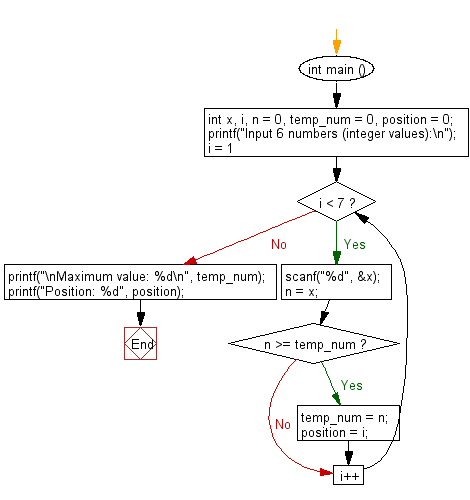﻿ C : Find the highest integer and its position from seven

# C Exercises: From seven integer values find the highest value and it's position

## C Basic Declarations and Expressions: Exercise-112 with Solution

Write a C program that reads seven integer values from the user and finds the highest value and its position.

Sample Solution:

C Code:

``````#include <stdio.h>

int main () {
// Declare variables
int x, i, n = 0, temp_num = 0, position = 0;

// Prompt user for input
printf("Input 6 numbers (integer values):\n");

// Loop to read and process 6 numbers
for (i = 1; i < 7; i++){

// Read an integer from user and store it in 'x'
scanf("%d", &x);

n = x; // Assign 'x' to 'n'

// Check if 'n' is greater than or equal to 'temp_num'
if (n >= temp_num){
temp_num = n; // Update 'temp_num' with the new maximum value
position = i; // Update 'position' with the current position
}
}

// Print the maximum value and its position
printf("\nMaximum value: %d\n", temp_num);
printf("Position: %d", position);

return 0; // End of program
}
``````

Sample Output:

```Input 6 numbers (integer values):
15
20
25
17
-8
35

Maximum value: 35
Position: 6
```

Flowchart:C programming Code Editor:

What is the difficulty level of this exercise?

Test your Programming skills with w3resource's quiz.

﻿